# Linial, Nathan

## Signing a graph to have small magnitude eigenvalues ★★

Author(s): Bilu; Linial

Conjecture   If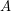is the adjacency matrix of a-regular graph, then there is a symmetric signing of(i.e. replace someentries by) so that the resulting matrix has all eigenvalues of magnitude at most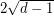.

Keywords: eigenvalue; expander; Ramanujan graph; signed graph; signing

## Linial-Berge path partition duality ★★★

Author(s): Berge; Linial

Conjecture   The minimum-norm of a path partition on a directed graph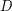is no more than the maximal size of an induced-colorable subgraph.

Keywords: coloring; directed path; partition

## The Alon-Tarsi basis conjecture ★★

Author(s): Alon; Linial; Meshulam

Conjecture   If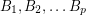are invertiblematrices with entries infor a prime, then there is asubmatrixof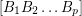so thatis an AT-base.

Conjecture   For every prime, there is a constant(possibly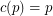) so that the union (as multisets) of anybases of the vector space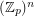contains an additive basis.